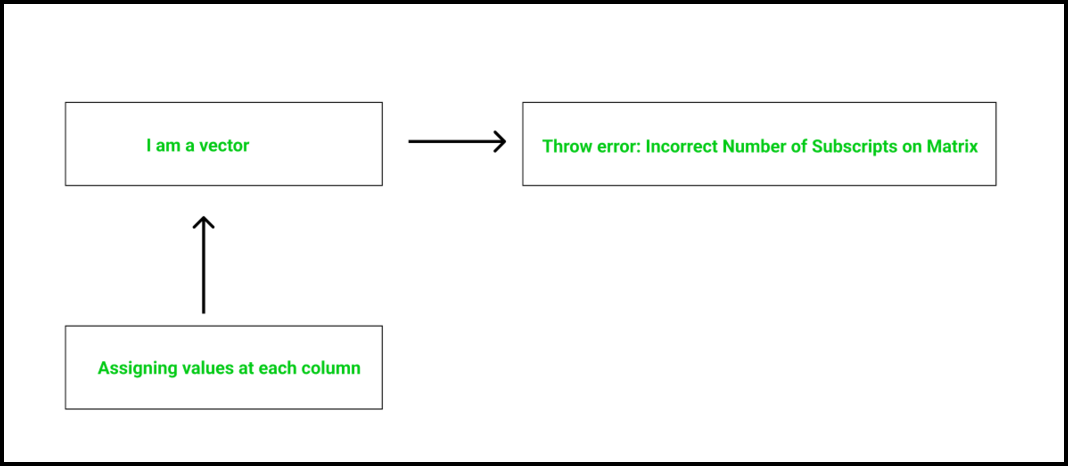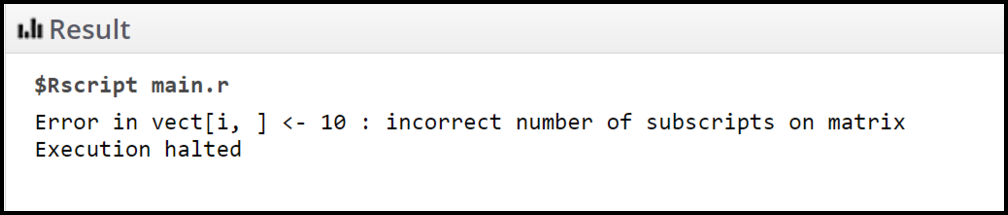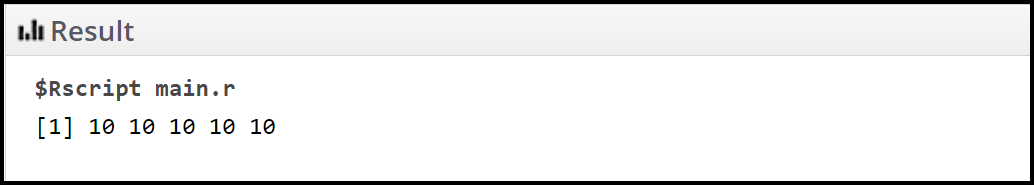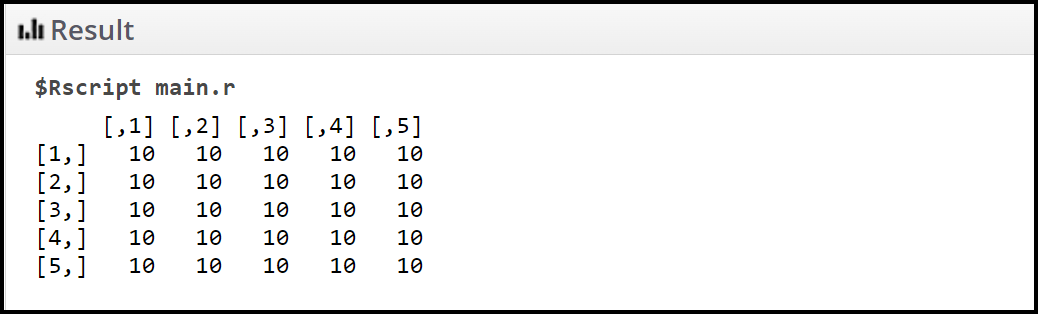# How to Fix: incorrect number of subscripts on matrix in R

• Last Updated : 21 Feb, 2022

Getting errors in programming is quite common and their frequency increase when our source code becomes bulkier. In this article, we are going to discuss how to fix: the incorrect number of subscripts on the matrix in R.

This error doesn’t give us any clue at the first appearance. This error may occur due to a very small mistake. This error may be easy for programmers to make but finding this error sometimes becomes challenging for most of the programmers.## When this error might occur?

These errors might occur when the programmer tries to use matrix notation on a data structure that is not a matrix on its own. This type of mistake is a normal one but sometimes it might be difficult to find if you don’t have any clarity regarding the data structure being used.

Example:

## R

 `# R program to illustrate when this  ` `# error might occur ` ` `  `# Creating a vector ` `vect = ``rep``(1, 5) ` ` `  `# Assign values in the created vector  ` `for``(i ``in` `1:5) ` `{ ` `  ``# Assigning at all columns of the  ` `  ``# ith row ` `  ``vect[i,] = 10 ` `}`

Output:## Why this error might occur?

This type of error occurs when the programmer tries to apply matrix notation to a vector.

Example:

In the below source code, we have created a vector vect (1 – dimensional data structure). Using a for-loop we are iterating from i = 1 to i = 100 and at each step of the iteration, we are assigning the value 10 to all columns of the ith row. But vect is a 1-dimensional data structure and we are applying matrix notation on it. Hence, the below source code will lead to an error: “the incorrect number of subscripts on the matrix” as clearly seen in the output.

## R

 `# R program to illustrate reasons for the error ` ` `  `# Creating a vector ` `vect = ``rep``(1, 5) ` ` `  `# Assign values in the created vector  ` `for``(i ``in` `1:5) ` `{ ` `  ``# Assigning at all columns of the ith row ` `  ``vect[i,] = 10 ` `}`

## How to fix this error?

Fixing this error is based upon two different ideologies that are discussed below.

Ideology 1: When we want to deal with the 1-dimensional data structure.

When we are sure that we are going to deal with a 1-dimensional data structure (like a vector) then we can fix this error like the below example.

Example:

## R

 `# R program to fix the error ` ` `  `# Creating a vector ` `vect = ``rep``(1, 5) ` ` `  `# Assign values in the created vector  ` `# Note that we have removed the comma (,) ` `# Since we are dealing with 1-dimensional  ` `# data structure ` `for``(i ``in` `1:5) ` `{ ` `  ``# Assigning 10 as the value ` `  ``# at the ith index of the vect  ` `  ``vect[i] = 10 ` `}`

Output:Ideology 2: When we want to deal with the 2-dimensional data structure.

When we are sure that we are going to deal with a 2-dimensional data structure (like a matrix) then we can fix this error like the below example.

Example:

## R

 `# R program to fix the error ` ` `  `# Creating a 2-dimensional data structure (matrix) ` `# mat has 5 rows and 5 columns  ` `mat = ``matrix``(``rep``(0,5), nrow=5, ncol=5) ` ` `  `# Assign values in the created matrix ` `# Note that we have added the comma (,) ` `# Since we are dealing with 2-dimensional ` `# data structure ` `for``(i ``in` `1:5) ` `{ ` `  ``# Assigning 10 as the value ` `  ``# at all columns of the ith row   ` `  ``mat[i,] = 10 ` `} ` ` `  `# Print the matrix ` `print``(mat)`

Output:My Personal Notes arrow_drop_up
Recommended Articles
Page :